# Reflected regions background¶

## Overview¶

This technique is used in classical Cherenkov astronomy for the 1D spectral extraction. A region on the sky, the ON region, is chosen to select events around the studied source position. In the absence of a solid template of the residual hadronic background, a classical method to estimate it is the so-called Reflected Region Background. The underlying assumption is that the background is approximately purely radial in the field-of-view. A set of OFF counts is found in the observation, by rotating the ON region selected around the pointing position. To avoid that the reflectd regions contain actual gamma-ray signal from other objects, one has to remove the gamma-ray bright parts of the field-of-view with a exclusion mask. Details on the reflected regions method can be found in [Berge2007]

The extraction of the ON and OFF events from the EventList of a set of observations is performed by the ReflectedRegionsBackgroundMaker. The latter uses the ReflectedRegionsFinder to create reflected regions for a given circular on region and exclusion mask.

## Using regions¶

The on region is a SkyRegion. It is typically a circle (CircleSkyRegion) for pointlike source analysis, but can be a more complex region such as a CircleAnnulusSkyRegion a EllipseSkyRegion, a RectangleSkyRegion etc.

The following example shows how to create such regions:

"""Example how to compute and plot reflected regions."""
import astropy.units as u
from astropy.coordinates import SkyCoord
from regions import CircleSkyRegion, EllipseAnnulusSkyRegion, RectangleSkyRegion
import matplotlib.pyplot as plt
from gammapy.maps import WcsNDMap

position = SkyCoord(83.63, 22.01, unit="deg", frame="icrs")

on_circle = CircleSkyRegion(position, 0.3 * u.deg)

on_ellipse_annulus = EllipseAnnulusSkyRegion(
center=position,
inner_width=1.5 * u.deg,
outer_width=2.5 * u.deg,
inner_height=3 * u.deg,
outer_height=4 * u.deg,
angle=130 * u.deg,
)

another_position = SkyCoord(80.3, 22.0, unit="deg")
on_rectangle = RectangleSkyRegion(
center=another_position, width=2.0 * u.deg, height=4.0 * u.deg, angle=50 * u.deg
)

# Now we plot those regions. We first create an empty map
empty_map = WcsNDMap.create(
skydir=position, width=10 * u.deg, binsz=0.1 * u.deg, proj="TAN"
)
empty_map.data += 1.0
empty_map.plot(cmap="gray", vmin=0, vmax=1)

# To plot the regions, we convert them to PixelRegion with the map wcs
on_circle.to_pixel(empty_map.geom.wcs).plot()
on_rectangle.to_pixel(empty_map.geom.wcs).plot()
on_ellipse_annulus.to_pixel(empty_map.geom.wcs).plot()

plt.show()


(png, hires.png, pdf)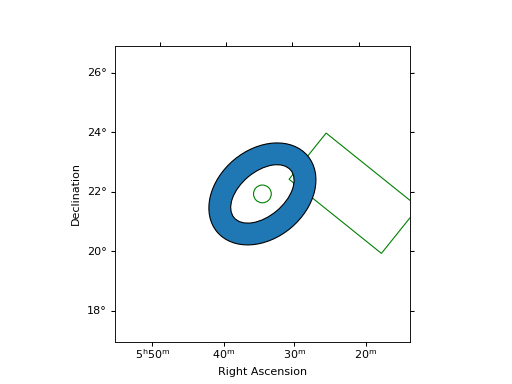## The reflected region finder¶

The following example illustrates how to create reflected regions for a given circular on region and exclusion mask using the ReflectedRegionsFinder. In particular, it shows how to change the minimal distance between the ON region and the reflected regions. This is useful to limit contamination by events leaking out the ON region. It also shows how to change the minimum distance between adjacent regions as well as the maximum number of reflected regions.

"""Example how to compute and plot reflected regions."""
import numpy as np
from astropy.coordinates import Angle, SkyCoord
from regions import CircleSkyRegion
import matplotlib.pyplot as plt
from gammapy.makers import ReflectedRegionsFinder
from gammapy.maps import WcsNDMap

# Exclude a rectangular region
exclusion_mask = WcsNDMap.create(npix=(801, 701), binsz=0.01, skydir=(83.6, 23.0))

mask = (Angle("23 deg") < coords.dec) & (coords.dec < Angle("24 deg"))

pos = SkyCoord(83.633, 22.014, unit="deg")
center = SkyCoord(83.633, 24, unit="deg")

# One can impose a minimal distance between ON region and first reflected regions
finder = ReflectedRegionsFinder(
region=on_region,
center=center,
)
finder.run()

fig1 = plt.figure(1)
finder.plot(fig=fig1)

# One can impose a minimal distance between two adjacent regions
finder = ReflectedRegionsFinder(
region=on_region,
center=center,
)
finder.run()
fig2 = plt.figure(2)
finder.plot(fig=fig2)

# One can impose a maximal number of regions to be extracted
finder = ReflectedRegionsFinder(
region=on_region,
center=center,
max_region_number=5,
)
finder.run()
fig3 = plt.figure(3)
finder.plot(fig=fig3)

plt.show()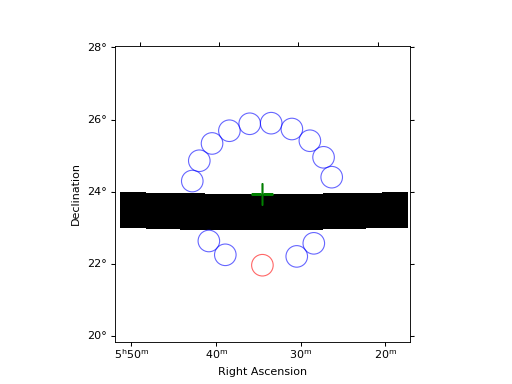(png, hires.png, pdf)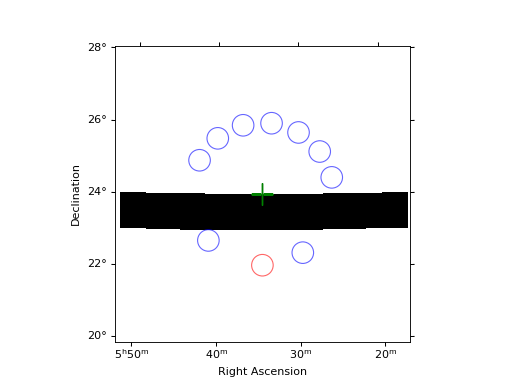(png, hires.png, pdf)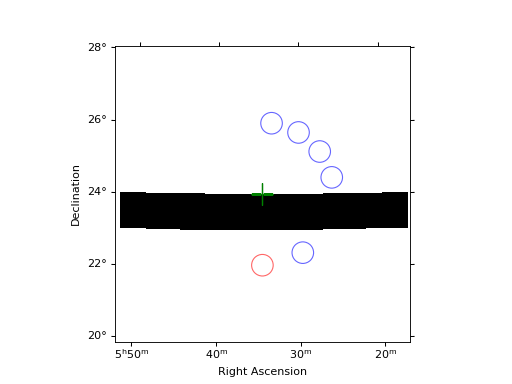(png, hires.png, pdf)

## Using the reflected background estimator¶

In practice, the user does not usually need to directly interact with the ReflectedRegionsFinder. This actually is done via the ReflectedRegionsBackgroundMaker, which extracts the ON and OFF events for an Observations object. The last example shows how to run it on a few observations with a rectangular region.

"""Example how to compute and plot reflected regions."""
import numpy as np
import astropy.units as u
from astropy.coordinates import SkyCoord
from regions import RectangleSkyRegion
import matplotlib.pyplot as plt
from gammapy.data import DataStore
from gammapy.datasets import SpectrumDataset
from gammapy.makers import ReflectedRegionsBackgroundMaker, SpectrumDatasetMaker
from gammapy.maps import Map, MapAxis
from gammapy.visualization import plot_spectrum_datasets_off_regions

data_store = DataStore.from_dir("\$GAMMAPY_DATA/hess-dl3-dr1/")
observations = data_store.get_observations(obs_ids)

crab_position = SkyCoord(83.63, 22.01, unit="deg", frame="icrs")

# The ON region center is defined in the icrs frame. The angle is defined w.r.t. to its axis.
rectangle = RectangleSkyRegion(
center=crab_position, width=0.5 * u.deg, height=0.4 * u.deg, angle=0 * u.deg
)

dataset_maker = SpectrumDatasetMaker(selection=["counts"])

e_reco = MapAxis.from_energy_bounds(0.1, 100, 30, unit="TeV")
dataset_empty = SpectrumDataset.create(e_reco=e_reco, region=rectangle)

datasets = []

for obs in observations:

dataset = dataset_maker.run(dataset_empty.copy(name=f"obs-{obs.obs_id}"), obs)
dataset_on_off = bkg_maker.run(observation=obs, dataset=dataset)
datasets.append(dataset_on_off)

m = Map.create(skydir=crab_position, width=(8, 8), proj="TAN")

_, ax, _ = m.plot(vmin=-1, vmax=0)

rectangle.to_pixel(ax.wcs).plot(ax=ax, color="black")

plot_spectrum_datasets_off_regions(datasets=datasets, ax=ax)
plt.show()


(png, hires.png, pdf)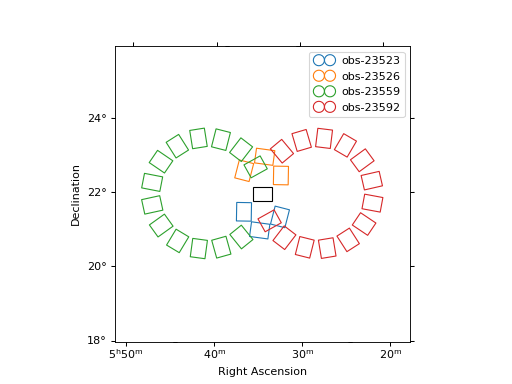The following notebook shows an example using ReflectedRegionsBackgroundMaker to perform a spectral extraction and fitting: